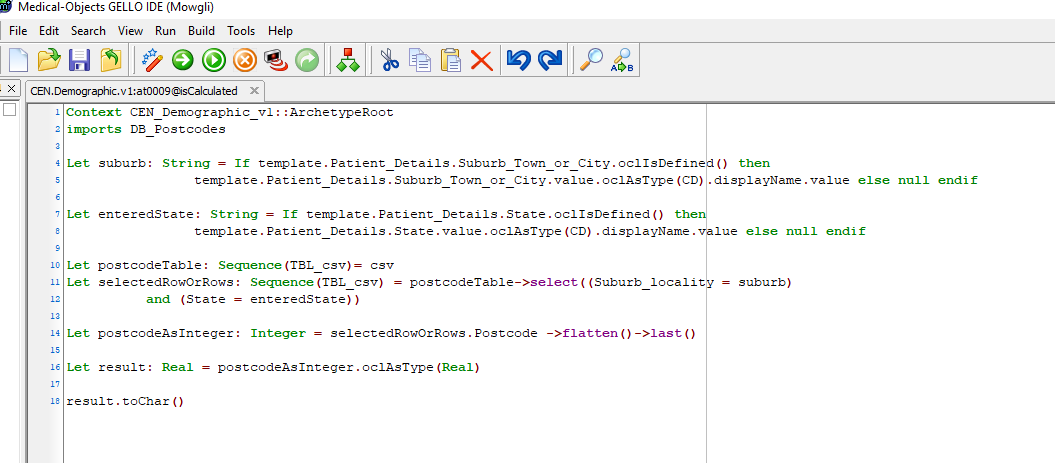GELLO can query a database. This example will show some some code that queries a database for a postcode based on a given locality. This page will then show it in use in an archetype.

Here is some code for this:

`imports DB_Postcodes`
`Let retrievedSuburbName: String = 'Bray Park' -- this will be the entered Suburb or localityLet retrievedStateName: String = 'NSW' -- this will be the entered State`
`Let postcodeTable: Sequence(TBL_csv)= csvLet selectedRowOrRows: Sequence(TBL_csv) = postcodeTable->select((Suburb_locality = retrievedSuburbName) and (State = retrievedStateName))`
`Let postcodeAsInteger: Integer = selectedRowOrRows.Postcode ->flatten()->last()`
`Let result: Real = postcodeAsInteger.oclAsType(Real)`
`result.toChar()`

DB_Postocdes in this case is a csv file. The MO Gello Authoring tool will also load SQL Lite file and Firebird files.The package presents as a sequence of rows. Here is a screen shot of the simple structure of this example: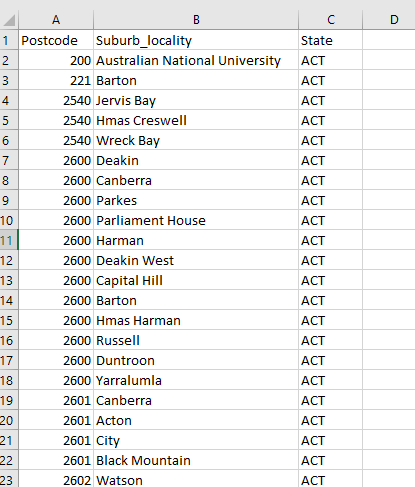This is the import screen: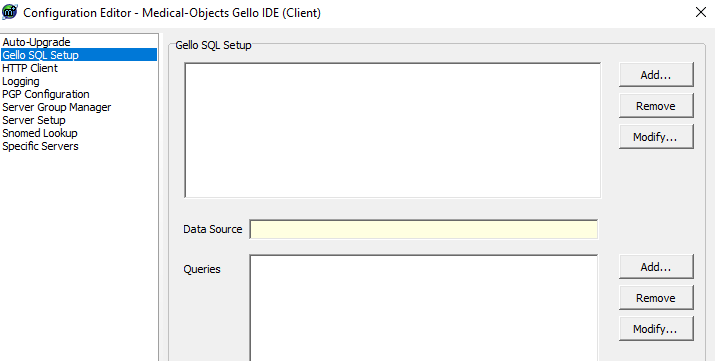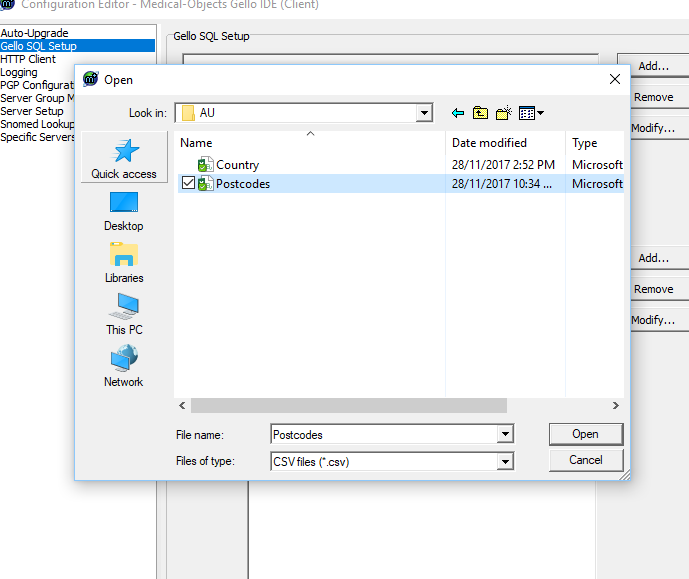Here is the code running: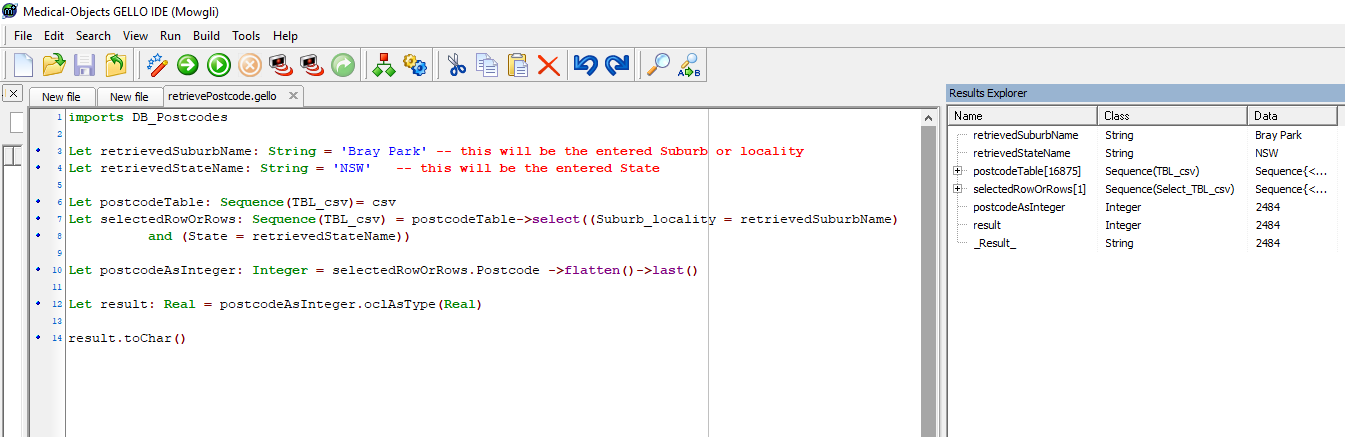The Suburb and the State can be captured from the UI of an archetype which runs this code in the onCalculate field for a Postcode Element:Here is the modified code that does this: